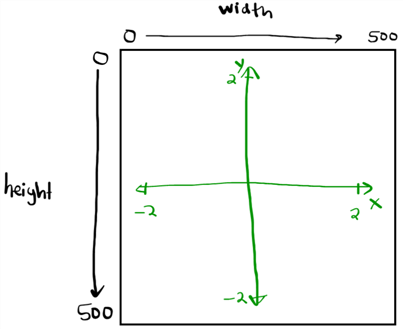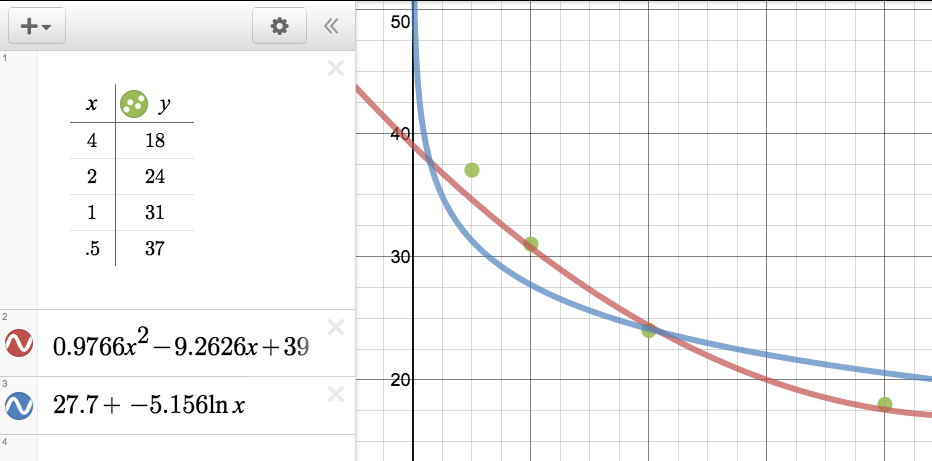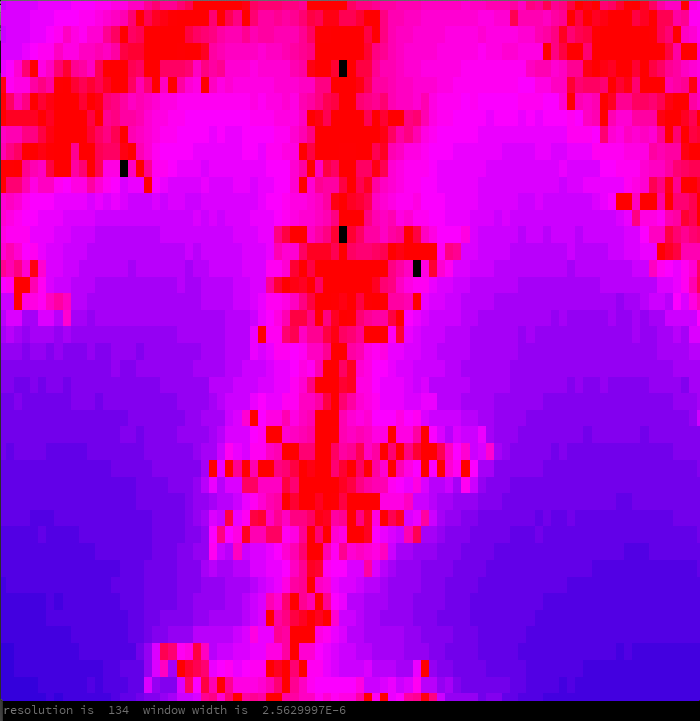# Mandelbrot Fractal v2

I had so much fun with the original Mandelbrot program that I decided to see if I could improve it. I was amazed by how easily I was able to generate a fractal picture that looked exactly like other pictures online. I didn’t look at anyone else’s code, just went down the path and the amazing Mandelbrot just happened.

Ok, now that you’re back: here are some new “features” that were implemented, and the interesting math that happened:

## Zooming

Left click to zoom in on that point (70% smaller window). Right click to zoom out ( 1/0.7 = 143% bigger window).

The first problem that I had with zooming was the conflict of the virtual coordinate complex plane (-2.0<x<2.0 and -2.0<y<2.0) and the processing.org window (0<width<500 pixels and 0<height<500 pixels, with the height pixels arranged from top to bottom (instead of bottom to top).After much brooding, lots of print(variable_name_here)s, lots of sketching on paper, and finally changing a + to a or  to a +, the zooming finally worked as desired. The code is more ugly than I’d normally like, but it’s generalized so you can change the resolution to whatever you’d like and it should work.
Here’s the processing code that takes a 1D pixel array (0 to 250,000) and produces each complex coordinate:

```  loadPixels();
color c;
float windowwidth = maxwindowx - minwindowx;
float windowheight = maxwindowy - minwindowy;
for (int i = 0; i < pixels.length; i++)
{
//this code maps the one dimensional pixel array to a cx and cy in the complex plane
float startcx = windowwidth * (float(i % width) / width) - (windowwidth/2) + centerx;
float startcy = windowheight * (float(floor(float(i)/width))/height) - (windowheight/2) + centery;```

The interesting math to get 2D information from a 1D array is here:

```i % width
floor(i / width)```

The first line gets the row (the % sign means remainder, so the 1052nd pixel is 1052 % 500 = 52, so it’s on row 52 (of 500)).
The second line gets the column (divides i and width, and rounds down, so floor(1052 / 500) = 2, so it’s on column 2.

The resolution is how the program calculates the color of each pixel.

Some background: the mandelbrot set is the set of all coordinates in the complex plane (x is reals, y is imaginary), c=a+bi, where if you start with the complex number z=0+0i, and iterate the following operation:
z := z^2 + c
The resultant complex number stays with a size (modulus) of less than or equal to 2. So in otherwords, if c = 0+0i, then z^2+c will always have a size of less than 2. Color this point black.

On the otherhand, if I start out with c=1+1i then I get the following sequence for z:

z_1 = (0+0i)^2+c = 1+1i  size <= 2, keep going.
z_2 = (1+1i)^2+c = 2i  size <= 2, keep going.
z_3 = (2i)^2+c = 1+3i  size > 2, stop.

So the complex point 1+1i fails after 3 steps. Color this point something dark because it fails somewhat quickly. If you make the rule that the longer a point goes before it fails, the lighter the color then you get something exactly like the above images. The coloring is that easy.

Here’s the coloring code:

```if  (mandelvalue < 2)
{
c = color(0,100,0);
}
else
{
//the color of the pixel depends on how far the iteration got before it was out of bounds
float ratio = 1.0*count/resolution;
c = color(10+1.0*ratio*140,100,1.0*ratio*200);
}```

If the final z (mandelvalue) is less than 2, then color it black, otherwise color it based on how many cycles it took to exceed two (count). Note: this color system is based on 0 to 100 HSB color format.

A big problem that has to be dealt with is when do you stop checking to see if a point converges to <= 2 or diverges….

Here’s a video of the resolution increasing one step at a time. A resolution of 1 means that all points whose size is <= 2 after 1 step are colored black. A resolution of 50 means that all points whose size is <= 2 after 50 steps are colored black. If the point strays outside of a size of 2, then color the point dependent on how quickly it strayed.

Ok. So clearly more resolution is better right? The problem is that it’s starting to get computationally slow. 500×500 is 250,000 pixels and at a resolution of 50 each one of these pixels may need up to 50 sets of calculations.

If we’re able to zoom in, then how can we make a clear enough picture for the given window size? If the picture is really zoomed in, then we’re going to need a higher resolution to get a nice picture.

So what I need is a way to dynamically change the resolution based on the virtual window size. If the virtual window size has a width of 4 units (-2 to 2), then a resolution of 18 is pretty good. But if the virtual window size has a width of 0.2 (-1.5 to -1.3) then I need a higher resolution. I need an equation to set the resolution based on the window size. Enter regressions. I took some points and made a quadratic regression:The x-axis is the windowwidth, and the y-axis is the resolution. The red graph was the first regression that I used. Not great. As the window got smaller and smaller, the increase in the resolution necessary didn’t keep up. So I went to a log regression (duh, vertical asymptote at x=0), and it picked a resolution with more accuracy.
Here’s the resulting code:

`resolution = int(27.71-5.156*log(windowwidth));`

## Varieties of Mandelbrot

z^2+c is only one possibility.
You can try z^n+c as well. Here’s a video walk through of z^2+c, z^3+c, z^4+c, … , z^10 + c. You can try this yourself in the real program by clicking the 2, 3, 4, 5, … , 9, and 0 (10) keys:

Here’s the code for the z^2 + c and the z^3 + c using binomial expansion that was done by hand (and simplification of powers of i).

```  if (power < 2.01 && power > 1.99)
{
fx = x*x - y*y + startcx;
fy = 2*x*y + startcy;
}
else if (power < 3.01 && power > 2.99)
{
fx = x*x*x-3*x*y*y + startcx;
fy = 3*x*x*y-y*y*y + startcy;
}```

But as I was going to wolframalpha to expand (a+bi)^4, I remembered DeMoivre’s Theorem. Duh. Here’s the general code:

```    float r = sqrt(x*x + y*y);
float theta = 0;
r = pow(r,power);
if (x > 0)
{
theta = atan(y/x);
}
else if (x<0)
{
theta = atan(y/x);
theta = theta + PI;
}
else
{
theta = 0;
}
theta = power * theta;
fx = r*cos(theta) + startcx;
fy = r*sin(theta) + startcy;```

This will work for any integer n for z^n+c. Great.
However this method is noticeably slower than the specific solutions. Bummer.

## Why Stick to Integer Powers?

I (now) know that DeMoivre's Theorem doesn't "work" for non-integer powers, but it sort of works.
Here's a video of the fractal walking from z^2+c to z^3+c by steps up in the power of 0.05. On the live version, you can try this yourself by clicking the "Q" key to go up by 0.05, and the "A" key to go down by 0.05. Notice the vertical line right down the middle? That's due to converting a rectangular point to polar and the program is trying to get pi/2 out of a theta of arctan(some_number / some_number_thats_really_really_small). See the trouble?

## Float Fail

Eventually we zoom too far down because the numbers are too small to accurately represent with a 32-bit float. Here's a picture of what happens when it eventually fails. At the bottom of the picture, I've printed out the resolution and the window width.A float only can store about 7 (decimal) digits of precision, so when we're squaring the same number 134 times, the errors will eventually catch up to us.

This entry was posted in Full Posts and tagged , , , . Bookmark the permalink.